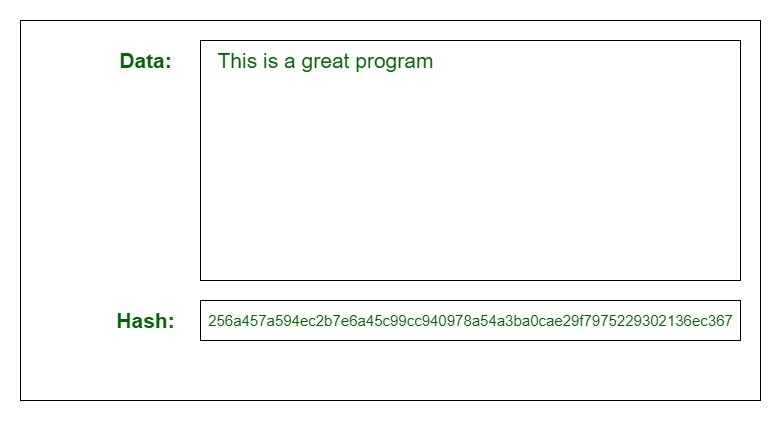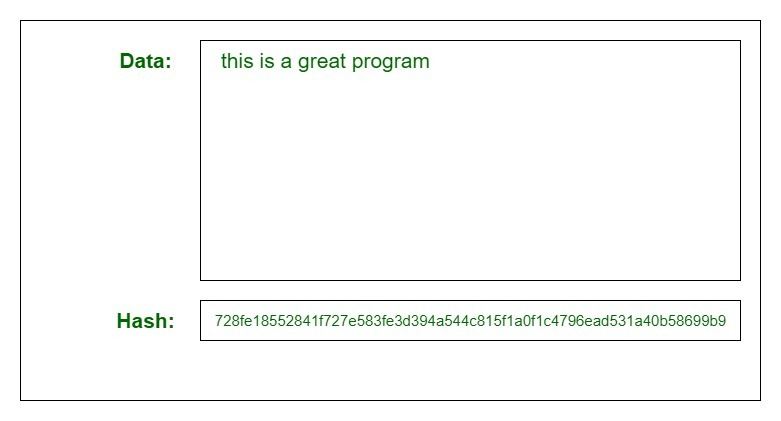## What is Hashing?

Hashing is the technique of encrypting the data inside the hashes using the hashtable. It uses various different techniques like CRC32, MD5, SHA256, etc.

Hashing is a technique that performs a search in constant time in order to perform insertion, deletion, finding. Hashing is the process of converting one value to another value. A hash function is used in the conversion.

In simple terms, Blockchain Hashing means taking an input string of any length and giving out an output of a fixed length. In the context of cryptocurrencies like bitcoin, the transactions are used as input and run through a hashing algorithm (Bitcoin uses SHA-256) which gives an output of a fixed length.## What is blockchain Hash?

In the blockchain, every block has a hash of the previous block. Blockchain hashing refers to the process of having an input item of whatever length reflecting an output item of a fixed length. If we take the example of blockchain use in cryptocurrencies, transactions of varying lengths are run through a given hashing algorithm, and all give an output that is of a fixed length. This is regardless of the length of the input transaction. The output is what we call a hash. A good example is Bitcoin’s Secure Hashing Algorithm 256 (commonly shortened to SHA-256). Hashing using SHA-256 always gives an output result of a fixed length, which has a 256-bits length (the output is 32 bytes).

This is always the case whether the transaction is just a single word or a complex transaction with huge amounts of data. What this means is that keeping track of a transaction becomes easier when you can recall/trace the hash. The size of the hash will depend on the hash function utilized, but the out using a particular algorithm will be of a specific size.Example:
When you make a video of say 500 megabytes and hash it using SHA-256, the output will be a hash of 256-bits in length. Similarly, if you take a text message of 50 kilobytes, the output hash will still be 256-bits. The only difference between the two will be the hash pattern. Let’s summarize the above information as follows: it is the umbrella term for Cryptographic hash functions

### Hashing process:

For this hash function, we can use Anders Brownworth Hash Program, a program developed by Anders Brownworth.If we type any character in the data section, we will observe its corresponding cryptographic hash in the hash section.Small Changes In The Input Changes the Hash:
If we make a small change in the input, the equivalent hash produced will be entirely different.Changing the capital “T” to small “t” completely changed the equivalent hash.

It is very difficult to determine the original string from the equivalent hash but it’s not impossible. The only method to determine the original string from its hash is by using “brute-force”. Brute-force basically means that we have to take random inputs, hash them, and compare them with the target hash.

There can be basically three scenarios:

1. Best case scenario:
We get our answer on the first try. The odds of this happening are astronomical.
2. Average case scenario:
In the case of SHA-256, we get our answer after 2^256/2 = 2^255 times. In other words, it’s a huge number.
3. Worst case scenario:
We get our answer at the end of the data.

Source:-

https://www.investopedia.com/terms/h/hash.asp#:~:text=A%20hash%20is%20a%20function,to%20blockchain%20management%20in%20cryptocurrency

https://www.onlinehashcrack.com/how-to-hashing-in-blockchain-explained.php

https://www.geeksforgeeks.org/blockchain-hash-function/

Comments are closed.

×

Powered by WhatsApp Chat

×